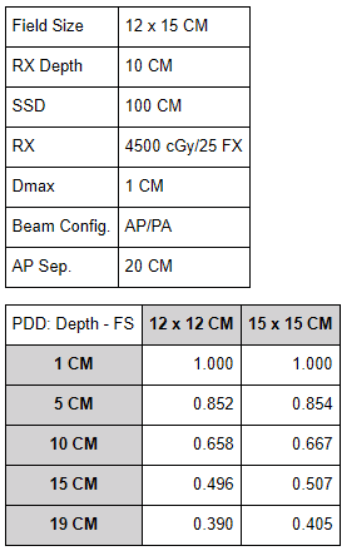# PDD Hand Calculation Guide

The following equations are used for PDD calculations:

• PDD = dose at depth (D_dx) / dose at dmax (D_dmax).
• Maynard’s factor = {[(SSD_2 + dmax) / (SSD_1 + dmax)] ^2} * {[(SSD_1 + depth) / (SSD_2 + depth)] ^2}.
• The Maynard’s factor is a conversion factor used in calculations when the SSD is greater than 100 CM.
• Equivalent Square = 4 * Area (A) / Perimeter (P).• Find the entrance dose at 1 CM depth
1. Calculate for equivalent square field size: 4*(12*15)/(12+12+15+15) = 13.3 x 13.3 CM
2. Interpolate for PDD at 10 CM depth w/ field size of 13.3 CM: {[(0.667-0.658)/30]*13}+0.658 = 0.662
3. Calculate for D_dmax: 90/D_dmax = 0.662; D_dmax = 135.95 cGy
4. Calculate for dose from AP beam to 1 CM depth: D_d1/135.95 = 1.00; D_d1 from AP = 135.95 cGy
5. Interpolate for PDD at 19 CM depth w/ field size of 13.3 CM: {[(0.405-0.390)/30]*13}+0.390 = 0.397
6. Calculate for dose from PA beam to 19 CM depth: D_d19/135.95 = 0.397; D_d19 from PA = 53.97 cGy
7. Find entrance dose at 1 CM depth: 135.95+53.97 = 189.92 cGy
• Find dose to the spinal cord at 15 CM depth
1. Interpolate for PDD at 15 CM w/ field size of 13.3 CM: {[(0.507-0.496)/30]*13}+0.496 = 0.501
2. Calculate for dose from AP beam to 15 CM depth: D_d15/135.95 = 0.501; D_d15 from AP = 68.11 cGy
3. Interpolate for PDD at 5 CM w/ field size of 13.3 CM: {[(0.854-0.852)/30]*13}+0.852 = 0.853
4. Calculate for dose from PA beam to 5 CM depth: D_d5/135.95 = 0.853; D_d5 from PA = 115.97 cGy
5. Find dose to the spinal cord at 15 CM depth: 68.11+115.97 = 184.08 cGy
• Find the entrance dose at 1 cm depth w/ SSD of 110 CM
1. Calculate for projected equivalent square field size at SSD of 110 CM: (13.3/100)*110 = 14.6 x 14.6 CM
2. Interpolate for PDD at 10 CM depth w/ projected field size of 14.6 CM = {[(0.667-0.658)/30]*26}+0.658 = 0.666
3. Calculate for Maynard’s factor at SSD of 110 CM for 10 CM depth: {[(110+1)/(100+1)]^2}*{[(100+10)/(110+10)]^2} = 1.015
4. Calculate for PDD at SSD of 110 CM to 10 CM depth w/ projected field size: 0.666*1.015 = 0.676
5. Calculate for D_dmax: 90/D_dmax = 0.676; Ddmax = 133.14 cGy
6. Interpolate for PDD at 1 CM depth w/ projected field size of 14.6 CM: {[(1.000-1.000)/30]*26}+1.000 = 1.000
7. Calculate for Maynard’s factor at SSD of 110 CM for 1 CM depth: {[(110+1)/(100+1)]^2}*{[(100+1)/(110+1)]^2} = 1.000
8. Calculate for PDD at SSD of 110 CM to 1 CM depth w/ projected field size: 1.000*1.000 = 1.000
9. Calculate for dose from AP beam to 1 CM depth: D_d1/133.14 = 1.000; D_d1 from AP = 133.14 cGy
10. Interpolate for PDD at 19 CM w/ projected field size of 14.6 CM: {[(0.405-0.390)/30]*26}+0.390 = 0.403
11. Calculate for Maynard’s factor at SSD of 110 CM for 19 CM depth: {[(110+1)/(100+1)]^2}*{[(100+19)/(110+19)]^2} = 1.028
12. Calculate for PDD at SSD of 110 CM to 19 CM depth w/ projected field size: 0.403*1.028 = 0.414
13. Calculate for dose from AP beam to 19 CM depth: D_d19/133.14 = 0.414; D_d19 from PA = 55.12 cGy
14. Find entrance dose at 1 CM depth w/ SSD of 110 CM: 113.14+55.12 = 168.26 cGy

Related Content: